Uh Oh! It seems you’re using an Ad blocker!

Since we’ve struggled a lot to makes online calculations for you, we are appealing to you to grant us by disabling the Ad blocker for this domain.# Empirical Rule Calculator

Calculation From

Mean

Standard Deviation

Dataset type

Enter Numbers (Separate Each No. by Comma)

Table of Content
 1 What is the empirical rule? 2 What is The Empirical Rule Formula? 3 What is The Percentage Rules? 4 How to Calculate Empirical Rule (Step-by-Step)/ 5 When do we use the Empirical Rule? 6 How do you use the empirical rule? 7 How do you use the empirical rule to solve problems? 8 What is the 69 95 99.7 rule? 9 Why is the empirical rule important? 10 What is 1sd 2sd 3sd? 11 Where did the empirical rule come from?
Get The Widget!

Add Empirical Rule Calculator to your website through which the user of the website will get the ease of utilizing calculator directly. And, this gadget is 100% free and simple to use; additionally, you can add it on multiple online platforms.

Available on App

This empirical rule calculator is an advanced tool to check the normal distribution of data within 3 ranges of standard deviation. Sometimes, this tool is also referred to as a three-sigma rule calculator or the 68 95 and 99.7 rule calculator. Just enter the mean and standard deviation if you select summary data or the sample or population if you select raw data to get the mean values for 68%, 95% and 99.7% of data within 3 SD ranges.

The empirical rule statistics calculator works on the basis of  68 95 99 rule statistics. Well, give a read to this article to know the empirical rule definition, the formula for the empirical rule, and an example of how to use this empirical calculator and empirical rule.

## What is the empirical rule?

The empirical rule implies that for a normal distribution almost all data lies within 3 standard deviations of the mean. According to this 68 95 99 rule, 68% of the data lies within the first standard deviation. Ninety-five percent of the data is to be kept within second standard deviations. While 99.7% of the data lies within third standard deviations. Empirical rule stats are briefly described as follows:

• Standard deviation is a measure of spread; it defines how much the data can change from the average, i.e., it shows the diversity of the dataset. The range of data is limited to smaller values.
• Symmetric distribution about the mean is the normal distribution, and the data that is near the mean occur more frequently than data that is far from the mean.
• Mean is the sum of available terms divided by the total number of terms.

While you’re dealing with a usual cattering of data, you can use this normal distribution empirical rule because of its ability to estimate probabilities. The empirical rule graph exhibits the three categories of the rule which are shown below: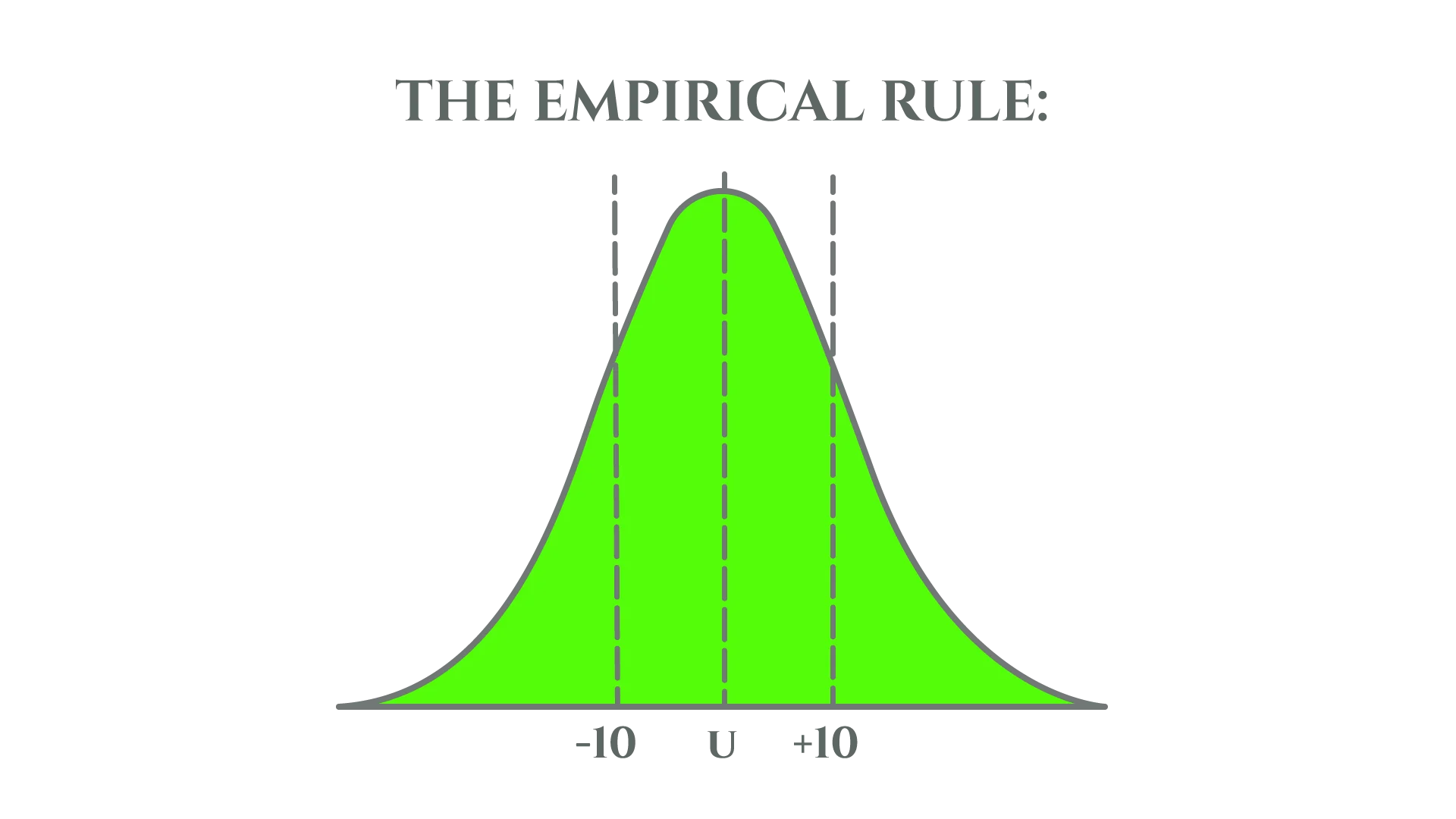### What is The Empirical Rule Formula?

From the given algorithm you will come to know about the formula, our empirical rule formula calculator also uses the same formula to calculate the normal distribution of data within 3 ranges of standard deviation.

Calculate the mean using: μ = (Σ xi) / n

• ∑ – indicates the sum of all given values
• xi – each value from the data
• N – total number of terms

Find the standard deviation using: σ = √ (∑ (xi – µ) ² / (n – 1))

The empirical rule formula is as follows:

• 68% of the data to be kept within 1 standard deviation from the mean – that is, the data lies between μ – σ and μ + σ.
• 95% of data lies within 2 standard deviations from the mean – between μ – 2σ and μ + 2σ.
• 99.7% of data lies among 3 standard deviations from the mean – between μ – 3σ and μ + 3σ.

To get the intervals all you have to do is to substitute the values of mean and standard deviation into this calculator for empirical rule.

### What is The Percentage Rules?

Empirical Rule is categorized into three percentages, 68, 95, and 99.7. therefore, it is also known as the 68 95 and 99.7 rule.

Different categories of the rule are:

• In a normal distribution, 68% of the data values will rest among 1 standard deviation (within 1 sigma) of the mean.
• In a normal, bell-shaped, distribution 95% of the data will fall into 2 standard deviations (within 2 sigma) of the mean.
• 99.7% of the data will be kept among 3 standard deviations (within 3 sigma) of the mean in a normal bell-shaped distribution. While the empirical rule percentages graph is given below representing the percentages accordingly: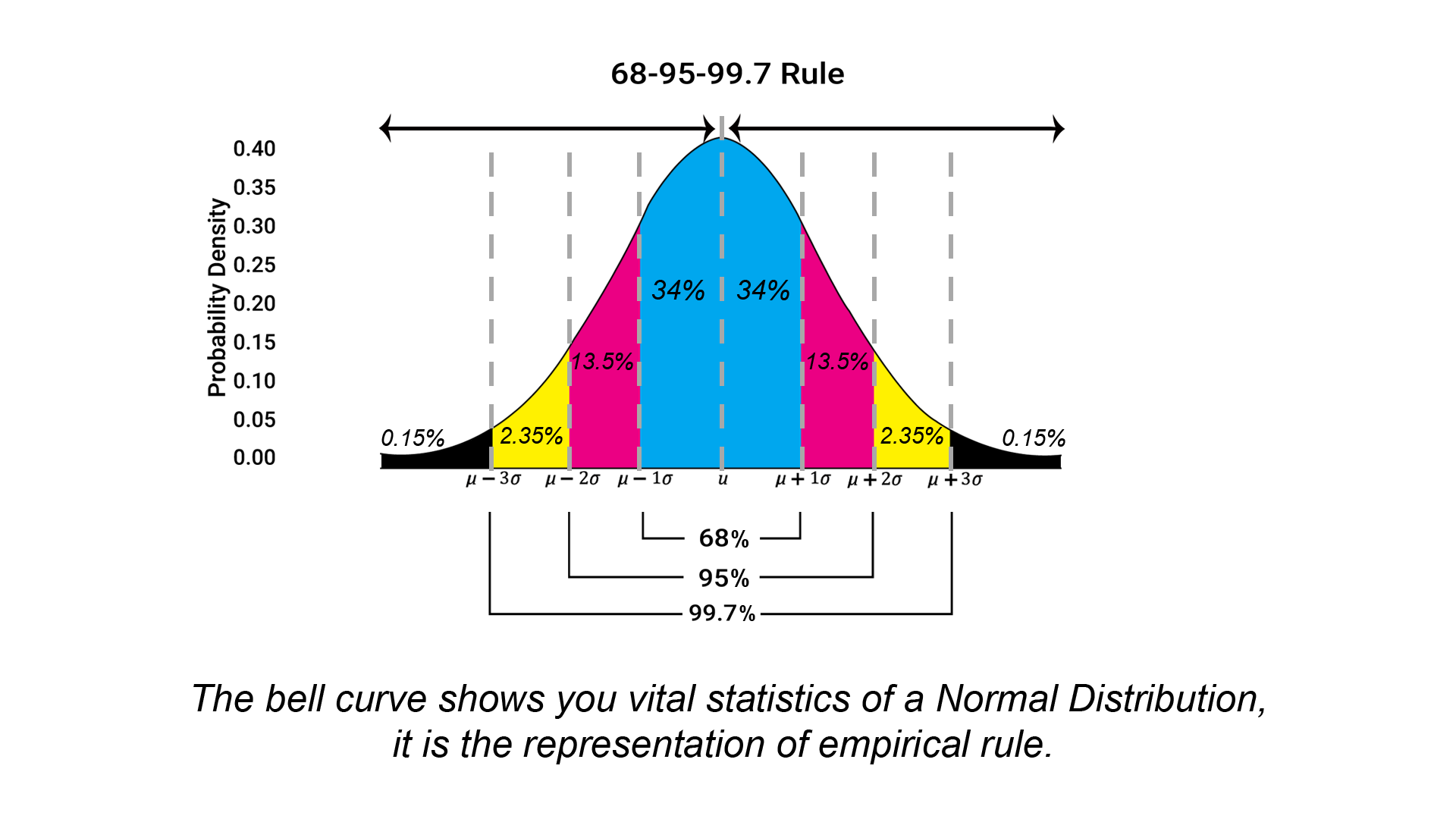## Bell Curve & Normal Distribution:

### Bell Curve:

The bell curve is a term that is typically used to describe the mathematical concept known as normal distribution, sometimes also referred to as Gaussian distribution. “Bell curve” is something that referred to as the bell shape that is created when a line is plotted by using the data points for an item that meets the criteria of normal distribution.
When it comes to bell curve, the center is consists of the greatest number of a value and, thus, it is said to be as the highest point the arc of the line. This is the point that is referred to as the mean, but in simple terms, it is said to be as the highest number of occurrences of an element (the mode, in statistical terms).

### Normal Distribution:

The immensely important thing you have to consider about a normal distribution is that the curve is concentrated in the center and decrease on either side. In normal distributions, the data has less of a tendency to produce unusually extreme values, said to be as outliers, as compared to other distributions. Also, the bell curve referred that the data is symmetrical.
Remember that the empirical rule came about because the same shape of distribution curves continued to appear over and over to statisticians. The 68 95 99.7 or three sigma rule applies to a normal distribution. When it comes to normal distribution, virtually all data lies within three standard deviations of the mean – The mean, mode, and medial all parameters are equal.
• The mean is referred to as the average of all of the numbers in the data set
• The mode is said to be as the number that repeats most frequently within the data set
• The median is said to be as the value of the spread between the highest and lowest numbers within the data set.
It indicates that the mean, mode, and median should all fall at the center of the dataset. You can note that half of the data should be at higher end of the data set, and the other half below.
A bell shape graph is based on two factors: the mean and the other is standard deviation. The mean is something that identifies the position of the center and the standard deviation computes the height and width of the bell. For instance, a large standard deviation makes a bell shape that is short and wide while a small standard deviation makes a tall and narrow curve shape.

This empirical rule calculator with graph supports you to find out if any specific data follows a normal distribution by checking if 68% of data fall within first standard deviation (σ), 95% of data fall within second standard deviation (σ) and 99.7% of data fall within first 3 standard deviations (σ). Whereas standard deviation, mean and normal distribution are empirical rule statistics.

The whole calculation follows the empirical rule for normal distribution.

### How to use the empirical rule calculator?

Our empirical rule formula calculator has two options, input, and the results. In the input section, there are two choices for the calculation form: (i) summary data and (ii) raw data. If you have the calculated mean and standard deviation, then you should select the first option (summary data). If you have not calculated the mean and standard deviation for your data, then select the second option (raw data). In the case of raw data, you just have to select the sample or population from the drop-down menu and enter the numbers. This tool will automatically apply the statistical empirical rule to give you the values for mean, standard deviation and the percentages of distributions.

#### To calculate a normal distribution for summary data:

If you have summary data, then you must select a ‘summary data’ option. In this case, you have the value of mean and standard deviation for your data. Follow the simple steps to check the data distribution.

Input

• From the “calculation form” just Select the summary data.
• Now enter the mean value of your data.
• Very next you have to enter the standard deviation.
• Hit the calculate button.

Output:

• The very first output will be your entered mean. It will remain the same and represented by (x̅)
• As a second output, you will have your value of standard deviation represented by (s)
• Values will be given for 68% data falls between the first standard deviation.
• Values will be given for 95% data falls between the second standard deviation.
• Values will be given for 99.7% data falls between the third standard deviation.
• Also, this bell shaped distribution calculator shows you the complete bell shaped empirical rule graph

#### To calculate a normal distribution for raw data:

If you have your data in sample or population then you need to select the raw data option from the drop-down menu. The calculator will do the rest.

Input:

• From the “calculation form” just Select the raw data.
• The first input is dataset type and you just have to Select the population or sample.
• Now enter numbers according to your data.
• Hit the calculate button

Output:

• The very first output will be your mean represented by (x̅)
• you will have your value of standard deviation represented by (s)
• Values will be given for 68% data falls between the first standard deviation.
• Values will be given for 95% data falls between the second standard deviation.
• Values will be given for 99.7% data falls between the third standard deviation.
• This 68-95-99 calculator will show the bell-shaped empirical rule corresponding to empirical rule statistics

## How to Calculate Empirical Rule (Step-by-Step)?

If the values of Standard Deviation and mean are known anyone can calculate the value of empirical rule using empirical rule formula. Down below is the empirical rule example to better understanding the method.

Empirical Rule Formula derivation:

• 68% of values lies => mean ± sd
• 95% of value lies => mean ± 2 sd
• 99.73% of value lies => mean ± 3 sd

Here;

• sd represents standard deviation for the value that is given
• Mean represents the Arithmetic Mean for the given values

Example:

Let’s dig a little deep and find empirical rule for the values {12,32,45,53,21,43}

Part 1:

The 1st step is to Calculate Mean

• mean = (12+32+45+53+21+43)/6
• mean = 206/6
• mean = 34.33

Part 2:

Then find the value for Standard Deviation

• SD(σ)=√ (1/ (6-1) ((12-34.33)2+(32-34.33)2+(45-34.33)2+(53-34.33)2+(21-34.33)2+(43-34.33)2))
• SD(σ)=√ (1/5((-22.34)2+(-2.33)2+(10.67)2+(18.67)2+(-13.34)2+(8.67)2))
• SD(σ)=√ (1/5((498.76) +(5.43) +(113.78) +(348.45) +(177.77) +(75.12)))
• SD(σ)=√ (243.87)
• SD(σ)=15.62

This Standard Deviation Calculator can be used to find Standard deviation as well.

Part 3:

• In the final step apply empirical rule percentages
• 68% of values lie within 18.7171 to 49.9496
• 95% of Values lie within 3.1009 to 65.5658
• 97.73% of values lie within -12.5154 to 81.182

The example explained above is to understand the concept of Empirical rule. While doing such tangled calculations on your own, we recommend you to use our empirical rule percentage calculator to verify your results.

## When do we use the Empirical Rule?

• In empirical research, the empirical rule is excessively used, for calculating the chance of a certain piece of data occurring, or when all data is not available the empirical rule formula or calculator help to predict the most possible outcomes.
• You can get a deep understanding of the characteristics of a population without going through the trouble of testing everyone and this also helps you determine whether a given data set is normally distributed or not.
• Another application of the formula is to help determine outliers (results that differ significantly from others) which can be the result of experimental errors.
• The Empirical Rule is most commonly used in statistics for forecasting, especially when getting accurate results or data is difficult or impossible to get.

## Relationship between Chebyshev’s theorem and the Empirical Rule:

The Empirical Rule stats is an estimate that can be used only to those data sets who have a bell-shaped relative frequency histogram. With the help of the empirical rule formula, it calculates the percentage of the measurements that fall within the range of 1, 2, and 3 standard deviations of the mean. Whereas the Chebyshev’s Theorem can be applied to all kinds of data sets even if the data is not normally distributed. It defines the smallest amount of the quantities that fall inside one, two, or more standard deviations of the mean.

## Empirical Rule: Notation!

Given below are the conditions that are always considered true while applying Empirical Rule Formula:

• Almost 68% of the data lies between one standard deviation of the mean (or between the mean – one times the standard deviation, and the mean + 1 times the standard deviation). μ ± 1σ is the mathematical representation of the previous statement.
• The second condition implies that 95% of the data lies between two standard deviations of the mean (or between the mean – 2 times the standard deviation, and the mean + 2 times the standard deviation). This can be mathematically represented as μ ± 2σ
• And almost 99.7% of the data lies between three standard deviations of the mean (or between the mean – three times the standard deviation and the mean + three times the standard deviation). Also represented mathematically as μ ± 3σ. Technically this is the notation that is used by the Empirical rule calculator.

## Account the Symmetry of the Bell Curve to Further Divide Up Percentages:

### First Part of the Empirical Rule:

When it comes to using the symmetry of the bell curve, the percentages may be broken down even further. For instance, the first part of the empirical rule is stated as 68% of the data values are lie within 1 standard deviation. That is from z = -1 to z = +1. You can see that the bell curve (normal distribution) is symmetrical on the right and left, look at the bell curve that half of 68%, or 34%, of the data values lie between z = -1 and z = 0, and the remaining half, 34%, lie from z = 0 to z = +1.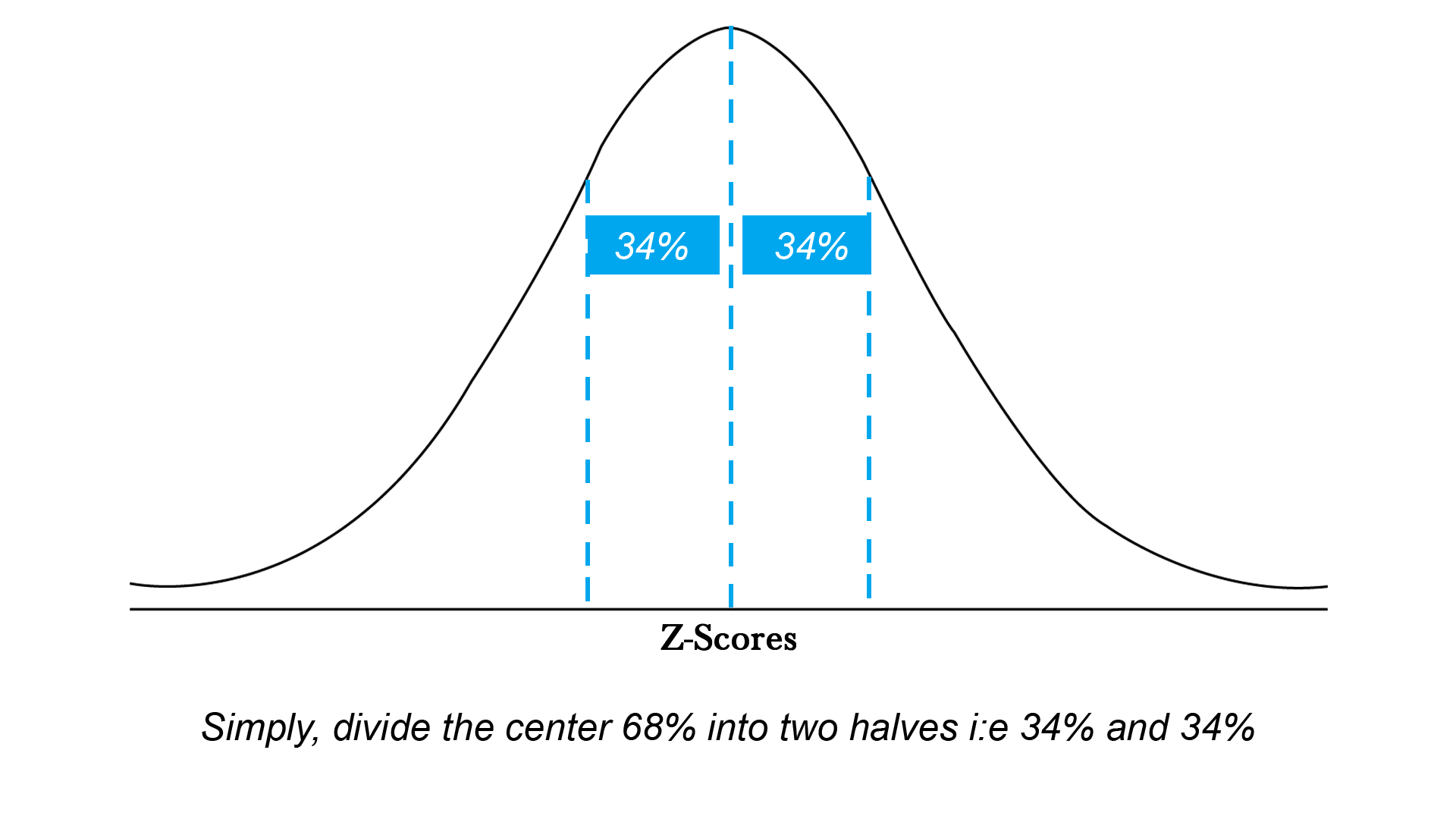Now, take a look at the second part of the empirical rule.

### Second Part of the Empirical Rule:

This part of empirical rule states that 95% of the data values are falls within 2 standard deviations i:e from z = -2 to z = +2. As the bell curve (normal distribution shape) is symmetrical on the right and left, you can notice that half of 95%, or 47.5% of the data values fall from z = -2 to z = 0, while other half that is 47.5% will lie from z = 0 to z = +2. So, by subtracting 34% from 47.5%, you can see that 13.5 of the data values fall from z = -2 to z = -1, while 13.5% of the data values are fall from z = +1 to z = +2. So, it’s time to expand the given diagram with that information.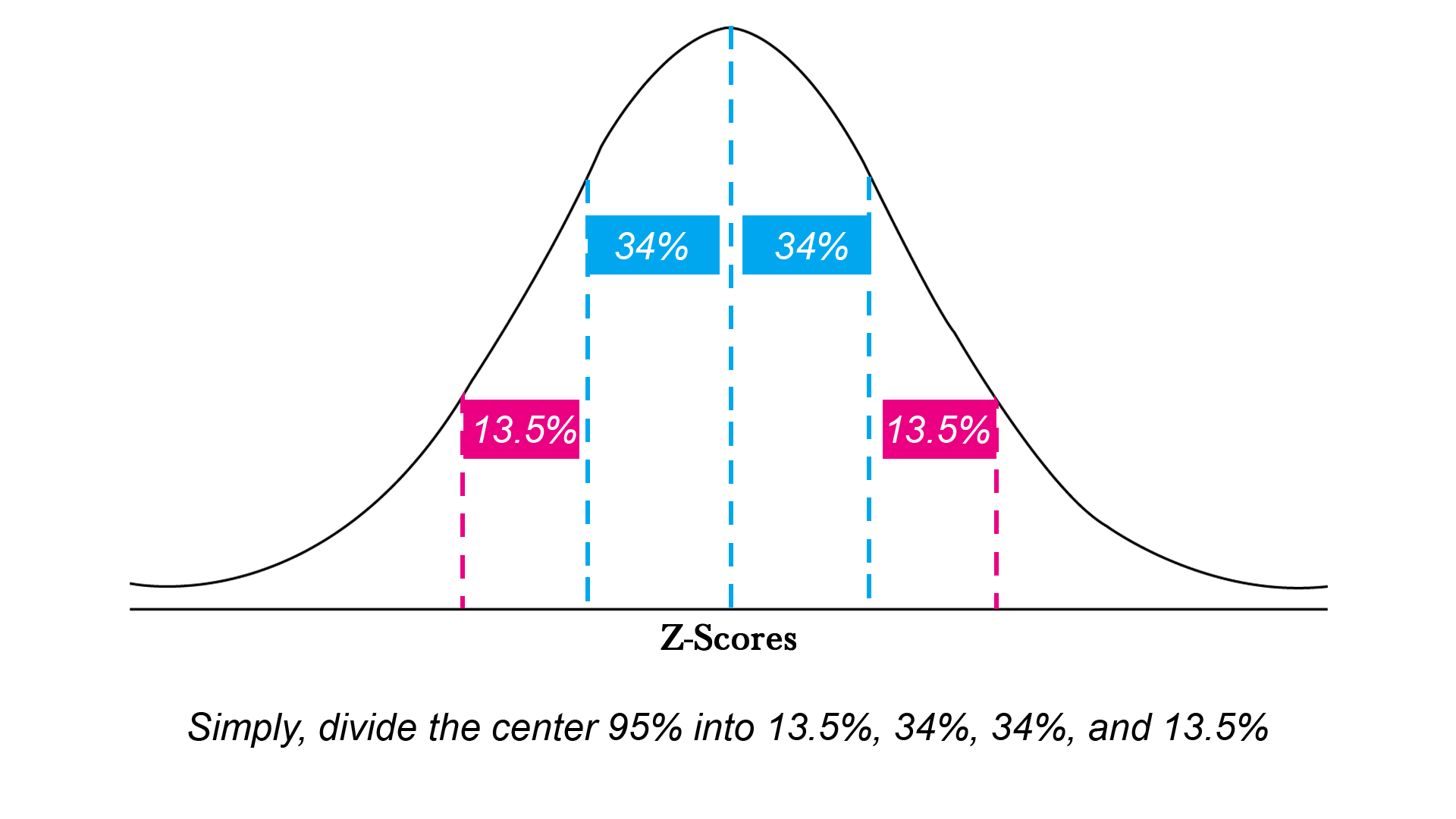### Third Part of the Empirical Rule:

Now, finally, take a look at the third part of the empirical rule.

The third part of the empirical rule states that 99.7% of the data values are lie within 3 standard deviations i: e from z = -3 to z = +3. While the % of area in the two remaining tails on the right and left should be 4.7%, as 99.7% – 95% is 4.7%. As the bell curve (normal distribution shape) is symmetrical on the left and right, you can note that half of 4.7%, or 2.35%, of the data values fall from z = -3 to z = -2, while the other half, 2.355 will lie from z = 2 to z = 3. So, expand the given diagram for better understanding.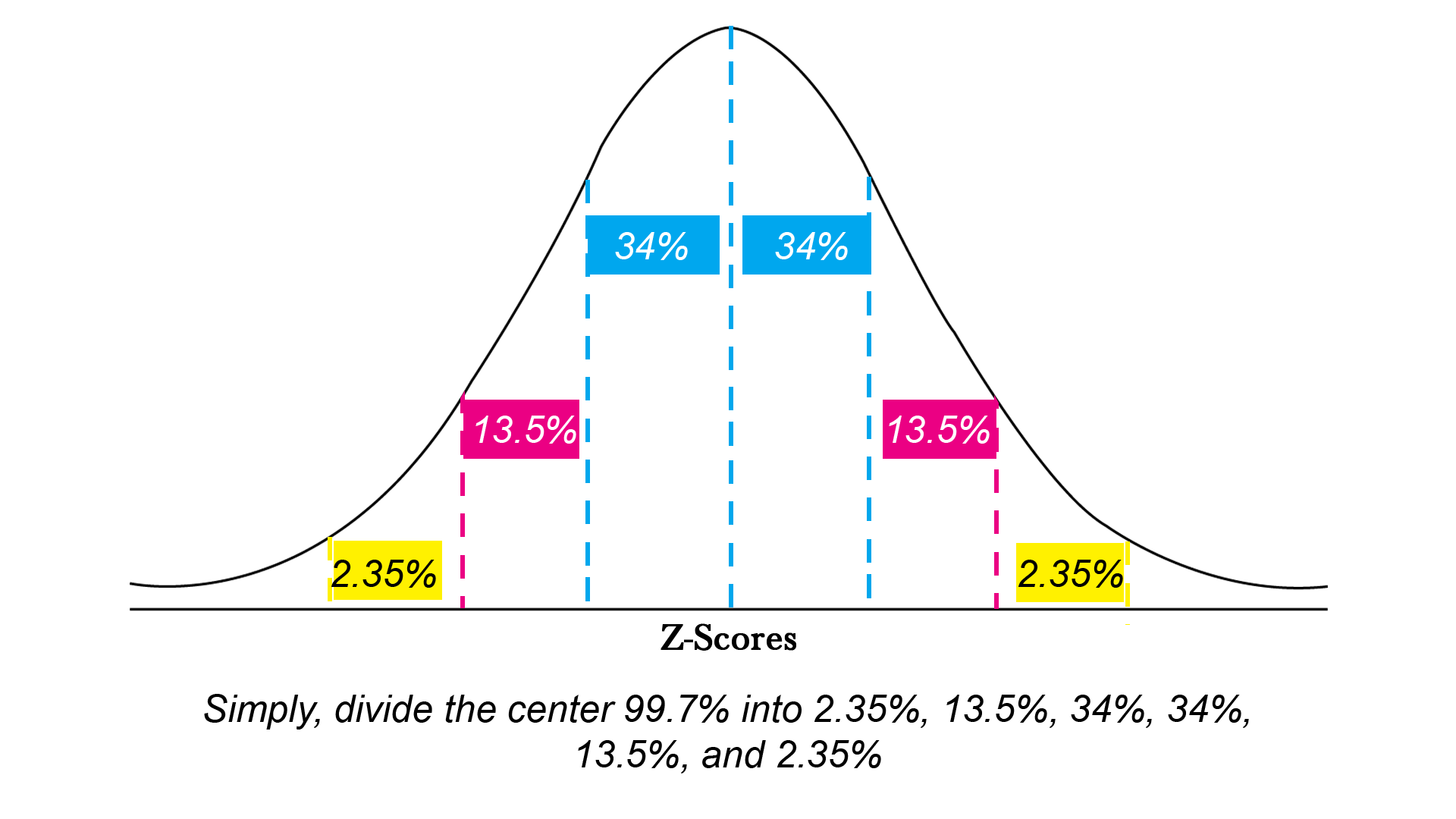## FAQ’s (Empirical Rule):

### How do you use the empirical rule?

This rule is an evaluation, according to a specific question precisely asks you to explain with the help of this Rule. while representing it you have to draw a normal curve with a line down the in the mid and three to both sides. Now simply write the values from the normal distribution.

### How do you use the empirical rule to solve problems?

Steps to Solving Empirical Rule Questions:

You have to take start with the values of mean, in the second step you have to add standard deviations. It will give you the values to the right. Now minus the standard deviations to get the values to the left. Write the percentages for each section. While doing it manually you have to memorize the values of relevant percentages.

### What is the 69 95 99.7 rule?

Empirical Rule is also known as 68-95-99.7 rule as according to it for any normal distribution, all of the data will fall within three standard deviations of the mean. Three SD are 68% 95% and 99.7%. this is the reason to call it 69 95 99.7 rule.

### How many standard deviations is 95th percentile?

If we assume a data set that represents an approximately normal distribution, the values within one standard deviation will be about 68% of the set while about 95% for 2SD and within three standard deviations values will be about 99.7%. in simple words it is a 95% probability.

### What is the range rule of thumb?

The rule states that the range is always four times greater than the standard deviation. Whereas the standard deviation is a measure of spread in statistics and plays a significant role in the empirical rule.

### Why is the empirical rule important?

It explains the pattern of distribution for any data set from the population. With the support of this rule, if you have only the mean and standard deviation of any normally distributed population, you will be able to calculate the chance or possibility of certain data occurring.

### What is the empirical framework?

Empirical research is grounded on experiential and measured occurrences and develops data from real understanding rather than from philosophy or faith. Researchers have to collect a real data. By finding values for mean and standard deviation empirical formula rule will be applied to assess the normal distribution probability.

### What is a Z-Score?

A Z-score also identified as a standard score. It provides an idea that how is the data point from the mean score. But more precisely it’s a term that explains how many standard deviations less or high then the mean score of any population.

Moreover, we can place it on a normal distribution curve.

### What is 1sd 2sd 3sd?

It means that 68% of values are less than one standard deviation represented by 1SD, 95% of values are less than two standard deviations represented by 2SD and 99% of values are less than three standard deviations represented by 3SD away from the mean respectively.

### What is the empirical rule formula in statistics?

According to this rule any normally distributed data, will always fall within three standard deviations of the mean. The rule can be divided into three parts: 68%,95%, and 97.5%.

### What is Chebyshev’s rule?

The Empirical Rule is a calculation for normally distributed data only whereas Chebyshev’s Theorem is that rule which can be easily applicable to all sort of data set. It refers to the least percentage of the measurements that fall within one, two, or more standard deviations of the mean.

### Where did the empirical rule come from?

The empirical rule originates as the same shape of distribution curves appeared again and again to statisticians. It signifies the normal distribution. So we can conclude that it came from the normal distribution.

### What normal distribution tells us?

The normal distribution is a possible distribution of the data. The percentage that falls below the curve within the two points on a distribution graph always points out the possibility that specific value will fall in that interval.

## Takeaway:

Manual calculations are always chaotic. Use this Empirical Rule Calculator as just by entering the values of mean and standard deviation you can easily find the percent of data values. If you don’t have a calculated mean and standard deviation, then enter your sample or population along with the relevant number and hit the calculate button. You will have the precise results. Additionally, you can use it as a learning tool.

From the source of investopedia – Understanding the Empirical Rule – By WILL KENTON – Example of The Empirical Rule

A Wiley Brand – Form dummies – Employing the Empirical Rule in Statistical Problems – Statistics Problems

From the source of sciencedirect – empirical rule statistics – Explore More By – Sheldon M. Ross, in Introductory Statistics (Third Edition) – Using Statistics to Summarize Data Sets – DESCRIPTIVE STATISTICS – By Sheldon M. Ross, in Introduction to Probability and Statistics for Engineers and Scientists (Fourth Edition)

By wikihow – Co-authored by wikiHow Staff – How to Use the Empirical Rule – Education and Communications – Studying – Subjects – Mathematics